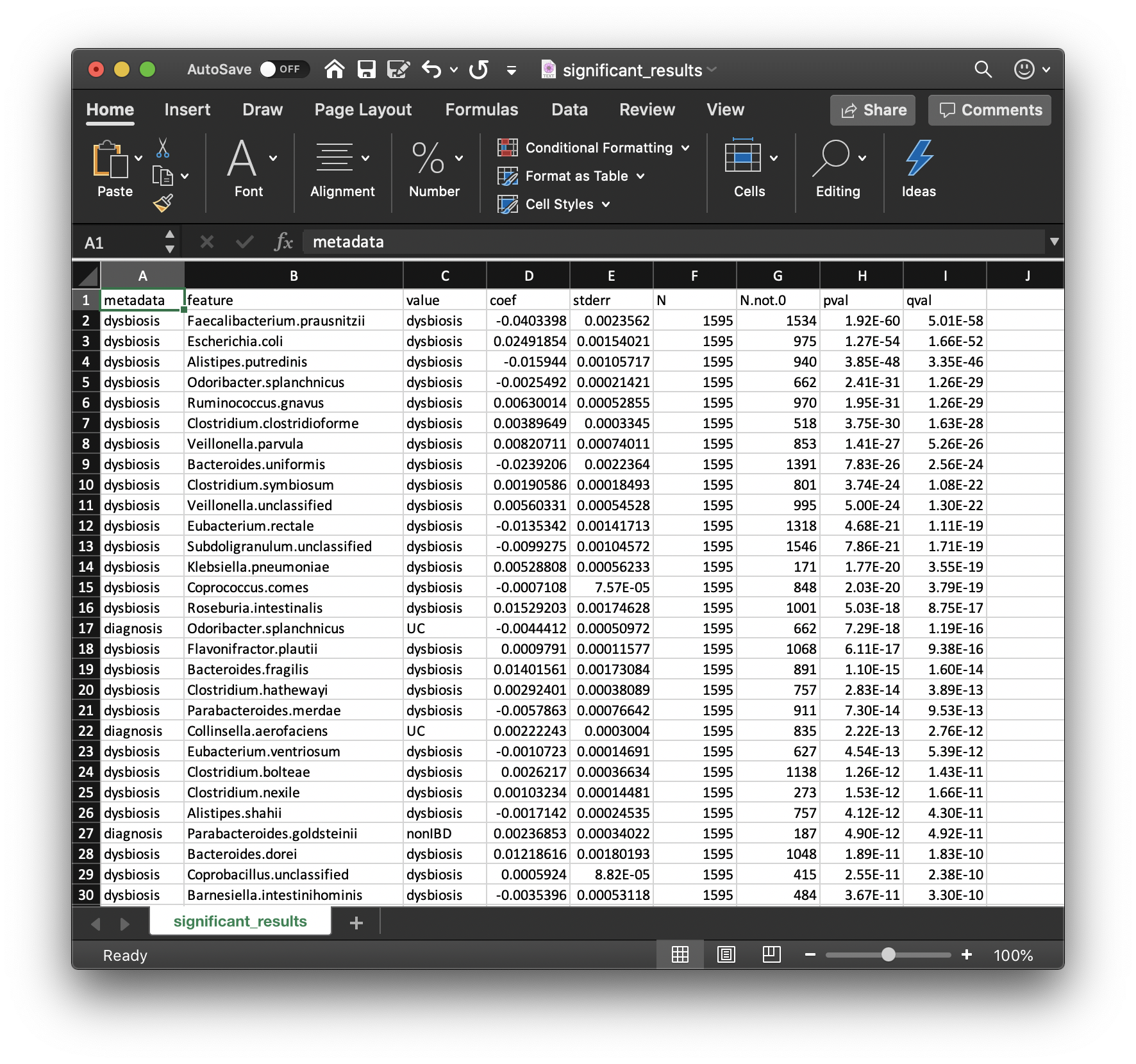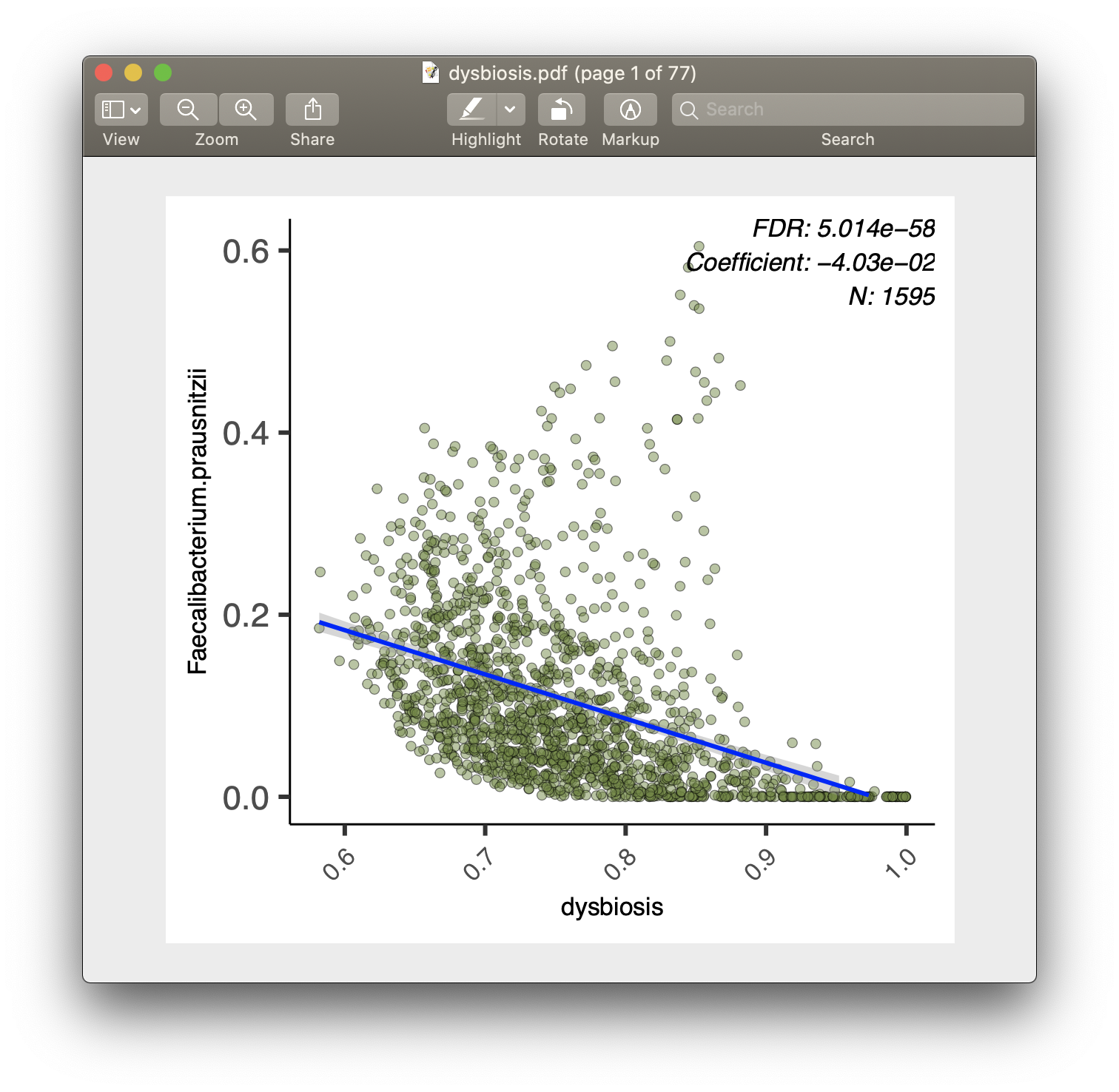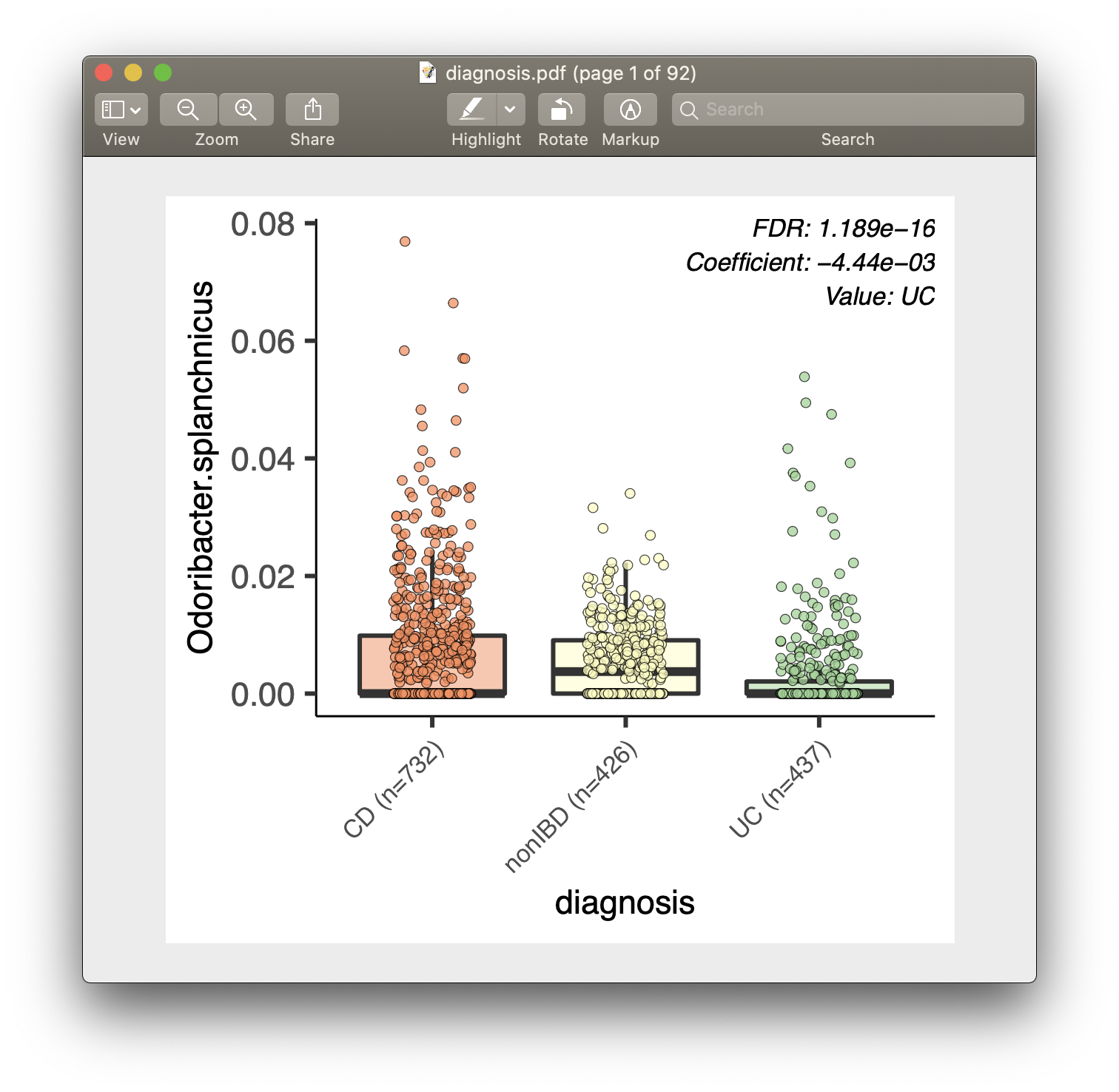## maaslin2

> maaslin2
MaAsLin2

MaAsLin2 is comprehensive R package for efficiently determining multivariable association between phenotypes, environments, exposures, covariates and microbial meta’omic features. MaAsLin2 relies on general linear models to accommodate most modern epidemiological study designs, including cross-sectional and longitudinal, and offers a variety of data exploration, normalization, and transformation methods.

For more information on the technical aspects:

User manual || Tutorial || Forum

Citation:

Mallick H, Rahnavard A, McIver LJ, Ma S, Zhang Y, Nguyen LH, Tickle TL, Weingart G, Ren B, Schwager EH, Chatterjee S, Thompson KN, Wilkinson JE, Subramanian A, Lu Y, Waldron L, Paulson JN, Franzosa EA, Bravo HC, Huttenhower C (2021). [Multivariable Association Discovery in Population-scale Meta-omics Studies]

PLoS Computational Biology, 17(11):e1009442

REQUIREMENTS
• R software
• R CRAN packages: pscl, pbapply, dplyr, vegan, chemometrics, ggplot2, pheatmap, cplm, logging, data.table, lmerTest
• R Bioconductor packages: edgeR, metagenomeSeq
GETTING STARTED

MaAsLin2 is an R package that can be run on the command line or as an R function. It requires the following R packages included in Biocondutor and CRAN (Comprehensive R Archive Network). Please install these packages before running MaAsLin2.

Installing MaAsLin2

With Bioconductor

Once you have the correct R version, you can install MaAsLin2 with Bioconductor:

``````if(!requireNamespace("BiocManager", quietly = TRUE))
install.packages("BiocManager")
BiocManager::install("Maaslin2")
``````

The `package ‘Maaslin2’ is not available` error.

You might encounter an error message that states:

``````Warning message:
package ‘Maaslin2’ is not available (for R version x.x.x)
``````

This can be due to one of two reasons:

1. Your R version is too old. Check this with `sessionInfo()` - if it is older than 3.6, download the most updated version per instructions above.
2. You have an older version of Bioconductor. MaAsLin2 requires Bioconductor >= 3.10. You can check your Bioconductor version with `BiocManager::version()`. If the returned version is anything older than 3.10, you can update with
`BiocManager::install(version = "3.10")`
3. And then `BiocManager::install("Maaslin2")`
Microbiome Association Detection with MaAsLin2
MaAsLin2 Input

Set up

Launch RStudio and in the R script window:

``````rm(list=ls())
getwd()
setwd("~/Desktop")
dir.create("R_Maaslin_tutorial") # Create a new directory
setwd("R_Maaslin_tutorial") # Change the current working directory
getwd() #check if directory has been successfully changed

#Load MaAsLin2 package into the R environment
library(Maaslin2)
?Maaslin2
``````

MaAsLin2 requires two input files, one for taxonomic or functional feature abundances, and one for sample metadata.

1. Data (or features) file
• This file is tab-delimited.
• Formatted with features as columns and samples as rows.
• The transpose of this format is also okay.
• Possible features in this file include microbes, genes, pathways, etc.
• This file is tab-delimited.
• Formatted with features as columns and samples as rows.
• The transpose of this format is also okay.

The data file can contain samples not included in the metadata file (along with the reverse case). For both cases, those samples not included in both files will be removed from the analysis. Also the samples do not need to be in the same order in the two files.

Example input files can be found in the `inst/extdata` folder of the MaAsLin2 source. The files provided were generated from the HMP2 data which can be downloaded from https://ibdmdb.org/ .

``````input_data = system.file(
"extdata", "HMP2_taxonomy.tsv", package="Maaslin2") # The abundance table file
input_data
"extdata", "HMP2_metadata.tsv", package="Maaslin2") # The metadata table file
``````

`HMP2_taxonomy.tsv`: is a tab-demilited file with species as columns and samples as rows. It is a subset of the taxonomy file so it just includes the species abundances for all samples.

`HMP2_metadata.tsv`: is a tab-delimited file with samples as rows and metadata as columns. It is a subset of the metadata file so that it just includes some of the fields.

Saving inputs as data frames

``````df_input_data = read.table(file = input_data, header = TRUE, sep = "\t",
row.names = 1,
stringsAsFactors = FALSE)
df_input_data[1:5, 1:5]
row.names = 1,
stringsAsFactors = FALSE)
``````
Running MaAsLin2

The following command runs MaAsLin2 on the HMP2 data, running a multivariable regression model to test for the association between microbial species abundance versus IBD diagnosis and dysbiosis scores (`fixed_effects = c("diagnosis", "dysbiosis")`). Output are generated in a folder called `demo_output` under the current working directory (`output = "demo_output"`).

``````fit_data = Maaslin2(
input_data = input_data,
output = "demo_output",
fixed_effects = c("diagnosis", "dysbiosis"))
``````
Providing data frames as input

If you are familiar with the data frame class in R, you can also provide data frames for `input_data` and `input_metadata` for MaAsLin2, instead of file names. One potential benefit of this approach is that it allows you to easily manipulate these input data within the R environment.

``````fit_data2 = Maaslin2(
input_data = df_input_data,
output = "demo_output2",
fixed_effects = c("diagnosis", "dysbiosis"))
``````
• Question: how would I run the same analysis, but only on CD and nonIBD subjects?
• Hint: try `?subset`
MaAsLin2 Output

#### Significant associations

Perhaps the most important output from MaAsLin2 is the list of significant associations. These are provided in `significant_results.tsv`:These are the full list of associations that pass MaAsLin2's significance threshold, ordered by increasing q-values. Columns are:

• `metadata`: the variable name being associated with a microbial feature.
• `feature`: the microbial feature (taxon, gene, pathway, etc.).
• `value`: for categorical features, the specific feature level for which the coefficient and significance of association is being reported.
• `coef`: the model coefficient value (effect size).
• Coefficients for categorical variables indicate the contrast between the category specified in `value` versus the reference category.
• MaAsLin2 by default sets the first category in alphabetical order as the reference. See 4.1 Setting Reference Levels on how to change this behavior.
• `stderr`: the standard error from the model.
• `N`: the total number of samples used in the model for this association (since e.g. missing values can be excluded).
• `N.not.0`: the total of number of these samples in which the feature is non-zero.
• `pval`: the nominal significance of this association.
• `qval`: the corrected significance is computed with `p.adjust` with the correction method (BH, etc.).
• Question: how would you interpret the first row of this table?

For each of the significant associations in `significant_results.tsv`, MaAsLin2 also generates visualizations for inspection (boxplots for categorical variables, scatter plots for continuous). These are named as "metadata_name.pdf". For example, from our analysis run, we have the visualization files `dysbiosis.pdf` and `diagnosis.pdf`:#### Full list of output files

MaAsLin2 generates two types of output files: data and visualization.

1. Data output files
• `significant_results.tsv`
• As introduced above.
• `all_results.tsv`
• Same format as `significant_results.tsv`, but include all association results (instead of just the significant ones).
• You can also access this table within R using `fit_data\$results`.
• `residuals.rds`
• This file contains a data frame with residuals for each feature.
• `maaslin2.log`
• This file contains all log information for the run.
• It includes all settings, warnings, errors, and steps run.
2. Visualization output files
• `heatmap.pdf`
• This file contains a heatmap of the significant associations.
• `[a-z/0-9]+.pdf`
• A plot is generated for each significant association.
• Scatter plots are used for continuous metadata.
• Box plots are for categorical data.
• Data points plotted are after normalization, filtering, and transform.
Setting Reference Levels

Since MaAsLin2 by default treats the first category in alphabetical order as the reference, the first way of setting reference levels is by prefixing the desired reference category with strings such as `"a_"`:

``````unique(df_input_metadata\$diagnosis)
"a_nonIBD"
fit_data3 = Maaslin2(
input_data = df_input_data,
output = "demo_output3",
fixed_effects = c("diagnosis_modified", "dysbiosis"))
``````

Alternatively, we can set the reference level by changing the categorical into a factor (see `?factor`), with its first level being the desired reference:

``````df_input_metadata\$diagnosis_modified = factor(df_input_metadata\$diagnosis,
levels = c("nonIBD", "CD", "UC"))
fit_data4 = Maaslin2(
input_data = df_input_data,
output = "demo_output4",
fixed_effects = c("diagnosis_modified", "dysbiosis"))``````
Interactions

Many statistical analysis are interested in testing for interaction between certain variables. Unfortunately, MaAsLin2 does not provide a direct interface for this. Instead, the user needs to create artificial interaction columns as additional `fixed_effects` terms. Using the above fit as an example, to test for the interaction between `diagnosis_modified` and `dysbiosis`, I can create two additional columns: `CD_dysbiosis` and `UC_dysbiosis` (since the reference for `diagnosis_modified` is `nonIBD`):

``````df_input_metadata\$CD_dysbiosis = (df_input_metadata\$diagnosis_modified == "CD") *
fit_data5 = Maaslin2(
input_data = df_input_data,
output = "demo_output5",
fixed_effects = c("diagnosis_modified", "dysbiosis", "CD_dysbiosis", "UC_dysbiosis"))``````
Random Effects

Certain studies have a natural "grouping" of sample observations, such as by subject in longitudinal designs or by family in family designs. It is important for statistic analysis to address the non-independence between samples belonging to the same group MaAsLin2 provides a simple interface for this through the parameter `random_effects`, where the user can specify the grouping variable to run a mixed effect model instead. For example, we note that HMP2 is a longitudinal design where the same subject (column `subject`) can have multiple samples. We thus ask MaAsLin2 to use subject as its random effect grouping variable:

``````fit_data6 = Maaslin2(
input_data = df_input_data,
output = "demo_output6",
fixed_effects = c("diagnosis_modified", "dysbiosis"),
random_effects = c("subject"))
``````

If you are interested in testing the effect of time in a longitudinal study, then the time point variable should be included in `fixed_effects` during your MaAsLin2 call.

• Question: intuitively, can you think of a reason why it is important to address non-independence between samples?
• Hint: imagine the simple scenario where you have two subject, one case and one control, each has two samples.
• What is the effective sample size, when samples of the same subject are highly independent, versus when they are highly correlated?

MaAsLin2 provide many parameter options for different data pre-processing (normalization, filtering, transfomation) and other tasks. The full list of these options are:

• `min_abundance`
• The minimum abundance for each feature [ Default: `0` ]
• `min_prevalence`
• The minimum percent of samples for which a feature is detected at minimum abundance [ Default: `0.1` ]
• `max_significance`
• The q-value threshold for significance [ Default: `0.25` ]
• `normalization`
• The normalization method to apply [ Default: `"TSS"` ] [ Choices: `"TSS"``"CLR"``"CSS"``"NONE"``"TMM"` ]
• `transform`
• The transform to apply [ Default: `"LOG"` ] [ Choices: `"LOG"``"LOGIT"``"AST"``"NONE"` ]
• `analysis_method`
• The analysis method to apply [ Default: `"LM"` ] [ Choices: `"LM"``"CPLM"``"ZICP"``"NEGBIN"``"ZINB"` ]
• `correction`
• The correction method for computing the q-value [ Default: `"BH"` ]
• `standardize`
• Apply z-score so continuous metadata are on the same scale [ Default: `TRUE` ]
• `plot_heatmap`
• Generate a heatmap for the significant associations [ Default: `TRUE` ]
• `heatmap_first_n`
• In heatmap, plot top N features with significant associations [ Default: `50` ]
• `plot_scatter`
• Generate scatter plots for the significant associations [ Default: `TRUE` ]
• `cores`
• The number of R processes to run in parallel [ Default: `1` ]
Prevalence and abundance filtering in MaAsLin2

Typically, it only makes sense to test for feature-metadata associations if a feature is non-zero "enough" of the time. "Enough" can vary between studies, but a 10-50% minimum prevalence threshold is not unusual (and up to 70-90% can be reasonable). Since we're already using a small, selected subset of demo data, we'll use `min_prevalence = 0.1` to test only features with at least 10% non-zero values. Note: this is the default parameter setting in MaAsLin2.

Similarly, it's often desirable to test only features that reach a minimum abundance threshold in at least this many samples. By default, MaAsLin2 will consider any non-zero value to be reliable, and if you've already done sufficient QC in your dataset, this is appropriate. However, if you'd like to filter more or be conservative, you can set a minimum abundance threshold like `min_abundance = 0.0001` to test only features reaching at least this (relative) abundance.

Putting these arguments together, we can run MaAsLin2 (and it'll go even faster!) using both:

``````fit_data7 = Maaslin2(
input_data = df_input_data,